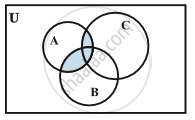# Operations on Sets - Intersection of Sets

#### definition

The intersection of two sets A and B is the set of all those elements which belong to both A and B. Symbolically, we write A ∩ B = {x : x ∈ A and x ∈ B}

#### notes

The intersection of sets A and B is the set of all elements which are common to both A and B. The symbol ‘∩’ is used to denote the intersection. The intersection of two sets A and B is the set of all those elements which belong to both A and B.
Example- A= {0,1,2,3} and B= {0,2,4,5}
Solution- A ∩ B= {0,2}

Some Properties of Operation of Intersection
a) Commutative law- The order of sequence to write the elements doesn't matter.
For instance, A ∩ B  = B ∩ A
b) Associative law- If you perform opertions on three or more elements then it doesn't matter how you group them, you will end up with same set.
For instance, ( A  ∩ B ) ∩ C = A ∩ ( B ∩ C )
c) Law of Ø and U- Intersection between a null set and anything will result in null set, whereas Intersection of a Universal set with anything results in that anything.
For instance,  Ø ∩ A = Ø, U ∩ A = A
d) Idempotent law- If we take intersection of two elements then result will be that single element because we are not taking anything new.
For instance, A ∩ A = A
e) Distributive law- This means intersection distributes over union.
For instance, A ∩ ( B ∪ C )   =  ( A ∩ B ) ∪ ( A ∩ C )If you would like to contribute notes or other learning material, please submit them using the button below.

### Shaalaa.com

Intersection of Sets [00:07:07]
S
0%